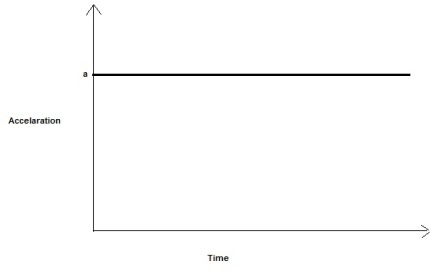# A toy car can move in either direction along a horizontal line (the + position axis). Assume the...

## Question:

A toy car can move in either direction along a horizontal line (the + position axis).

Assume the friction is so small that it can be ignored. A force toward the right of constant magnitude is applied to the car.

Sketch on the axes using a solid line the shape of the acceleration-time graph of the car.

## Newton's Second Law

According to Newton's second law of motion, the net force on an object is equal to mass times the acceleration of the object. If the net force on a object is constant, then the acceleration of the object is also constant.

## Answer and Explanation:

Let a constant horizontal force F is applied on the car of mass m

The horizontal acceleration of the car will be given by, {eq}a = \dfrac{F}{m} {/eq}

As force is constant, therefore, the acceleration of the car will also be constant.

The acceleration-time graph will be as follows,#### Learn more about this topic:Newton's Second Law: Meaning & Calculations

from Physics 111: Physics I

Chapter 6 / Lesson 7
2.2K